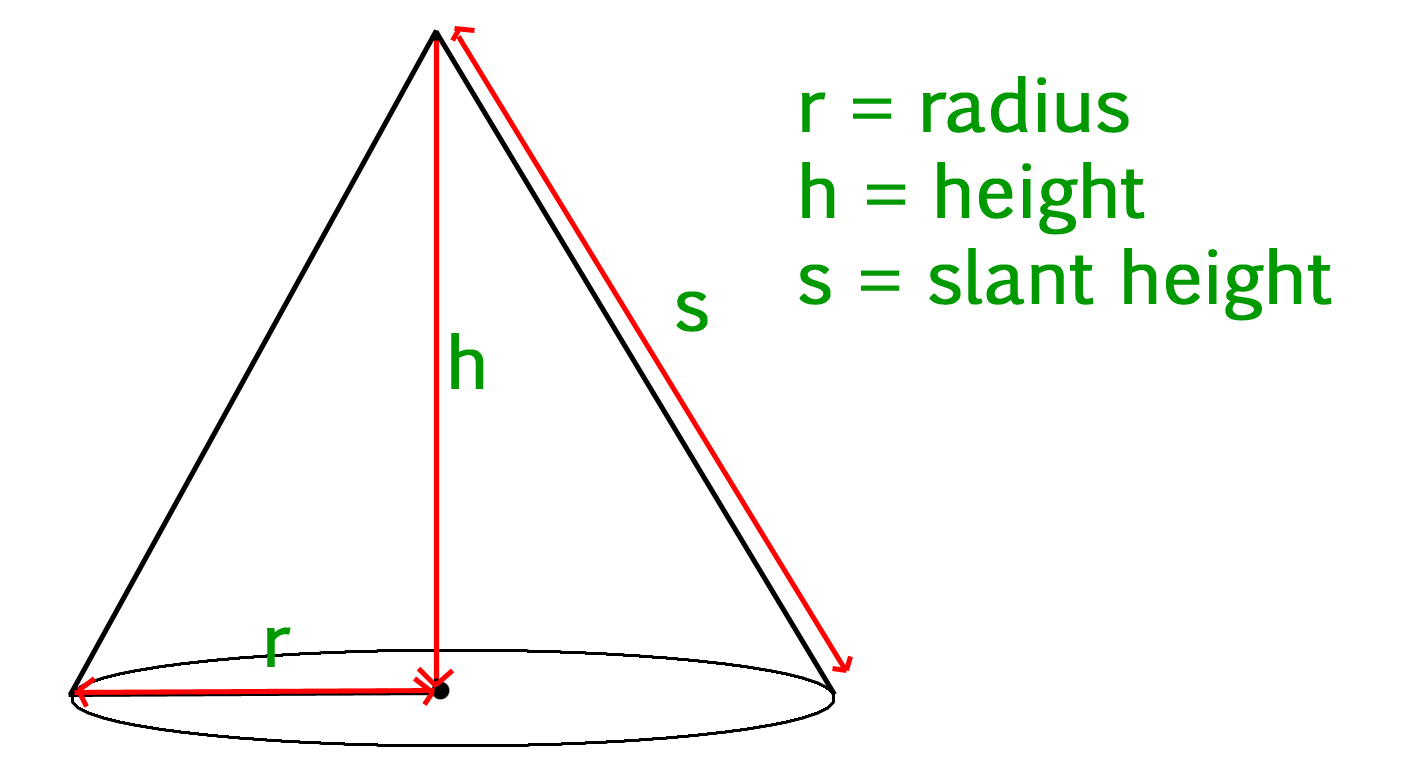Calculate volume and surface area of a cone

• Difficulty Level : Easy
• Last Updated : 24 Nov, 2021

Given slant height, height and radius of a cone, we have to calculate the volume and surface area of the cone.

• Cone :
Cone is a three dimensional geometric shape. It consists of a base having the shape of a circle and a curved side (the lateral surface) ending up in a tip called the apex or vertex.• Volume of a cone :
The volume of a cone is given by the formula –

volume = 1/3(pi * r * r * h)
• where r is the radius of the circular base, and h is the height (the perpendicular distance from the base to the vertex).

• Surface area of a cone :
The surface area of a cone is given by the formula –

area = pi * r * s + pi * r^2
• Where r is the radius of the circular base, and s is the slant height of the cone.

Examples :

Input :
slant_height = 13
height = 12
Output :
Volume Of Cone = 314.159
Surface Area Of Cone = 282.743

Input :
slant_height = 10
height = 8
Output :
Volume Of Cone = 301.593
Surface Area Of Cone = 301.593

C++

 // CPP program to calculate Volume // and Surface area of Cone#includeusing namespace std;  float pi = 3.14159;  // Function to calculate// Volume of conefloat volume(float r, float h){    return (float(1) / float(3)) * pi *                              r * r * h;}  // Function to calculate// Surface area of conefloat surface_area(float r, float s){    return pi * r * s + pi * r * r;}  // Driver Codeint main(){    float radius = 5;    float slant_height = 13;    float height = 12;    float vol, sur_area;      // Printing value of volume     // and surface area    cout << "Volume Of Cone : "        << volume(radius, height) << endl;    cout << "Surface Area Of Cone : "        << surface_area(radius, slant_height);    return 0;}

Java

 // Java program to calculate // Volume and Surface area of coneclass GFG {    static float pi = 3.14159f;      // Function to calculate     // Volume of cone    public static float volume(float r,                                float h)    {        return (float)1 / 3 * pi * h *                               r * r;    }      // Function to calculate     // Surface area of cone    public static float surface_area(float r,                                      float s)    {        return pi * r * s + pi * r * r;    }      // Driver Code    public static void main(String args[])    {        float radius = 5;        float slant_height = 13;        float height = 12;        float vol, sur_area;          // Printing value of volume        // and surface area        System.out.print("Volume Of Cone : ");        System.out.println(volume(radius, height));          System.out.print("Surface Area Of Cone : ");        System.out.println(surface_area(radius,                                         slant_height));              }}  // This code is contributed by "akanshgupta"

Python

 ''' Python3 program to calculate Volume and     Surface area of Cone'''  # Importing Math library for value Of PIimport mathpi = math.pi  # Function to calculate Volume of Conedef volume(r, h):    return (1 / 3) * pi * r * r * h  # Function To Calculate Surface Area of Conedef surfacearea(r, s):    return pi * r * s + pi * r * r  # Driver Coderadius = float(5)height = float(12)slat_height = float(13)print( "Volume Of Cone : ", volume(radius, height) )print( "Surface Area Of Cone : ", surfacearea(radius, slat_height) )

C#

 // C# program to calculate // Volume and Surface area of coneusing System;  class GFG {    static float pi = 3.14159f;      // Function to calculate     // Volume of cone    public static float volume(float r,                                float h)    {        return (float)1 / 3 * pi * h *                                r * r;    }      // Function to calculate     // Surface area of cone    public static float surface_area(float r,                                      float s)    {        return pi * r * s + pi * r * r;    }      // Driver Code    public static void Main()    {        float radius = 5;        float slant_height = 13;        float height = 12;        //float vol, sur_area;          // Printing value of volume         // and surface area        Console.Write("Volume Of Cone : ");        Console.WriteLine(volume(radius,                                  height));          Console.Write("Surface Area Of Cone : ");        Console.WriteLine(surface_area(radius,                                        slant_height));              }}  // This code is contributed by "vt_m"



Javascript



Output :

Volume Of Cone : 314.159
Surface Area Of Cone : 282.743

My Personal Notes arrow_drop_up